# How to split number into each individual digit in columns in Excel?

If you want to split a number with more than one digits into each individual digit in each column, what you can do? I think you can use the combination of MID and COLUMN functions to resolve your issue. Please see the method below in this article.

Let’s study the two functions first.

#1 MID function is used for capturing a specified length of characters from a string.

The formula is `= MID(text, start_num, num_chars)`.

#2 COLUMN function is used for returning the column number refer to the entering reference.

The formula is `= COLUMN(reference)`

COLUMN() without any reference returns the current column number for the selected cell. See example below.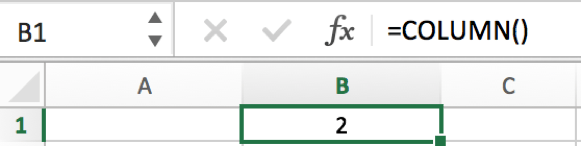Then we can start to learn how can we split a number into each individual digit.

#1 First, prepare a number like `12345678`. We can try to cut the first digit from this number. Enter` =MID(A2,1,1)` in B2 for example. A2 is the cell for number, 1 is the start position, 1 is the length of digit. We get the result below.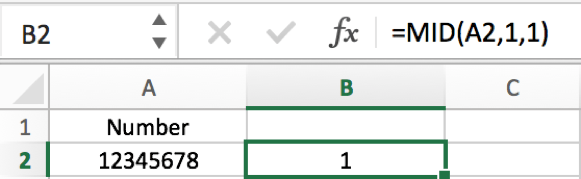#2 For MID function, the second parameter ‘start_num’, we can use COLUMN function to replace manual entering the start position. For example, we can enter `=MID(A2,COLUMN(),1)` in B2 to get the split digit.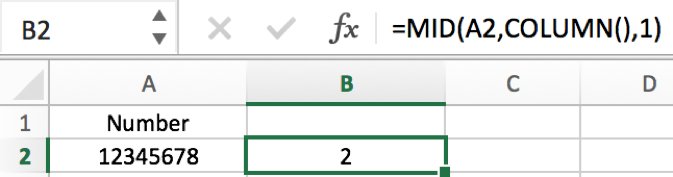Verify that we get the returned value 2. That’s because COLUMN() returns the current column number, and the selected cell is B2, so the MID function equals to `=MID(A2,2,1)`, so the returned value is 2.

#3 If we want to get eight individual digits from number 12345678 and put them into column B2 to I2. How can we do it? In above sample we can know COLUMN() returns 2 for B2, so if we want to get the first digit from number 12345678 into B2, we need to get return value 1, so we can use COLUMN()-1 as start number criteria.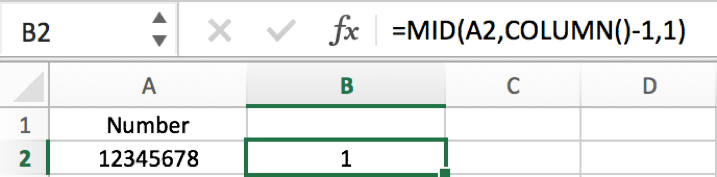#4 In cell C2 we need to get the second individual digit from number in A2, so we add \$ before A2 in formula =MID(\$A2,COLUMN()-1,1), in this case we can directly drag B2 and use this formula to fill C2:I2.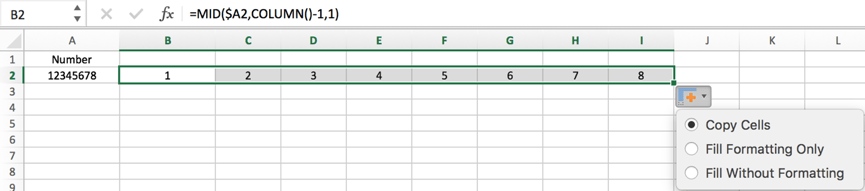Notes, if we still use formula =MID(A2,COLUMN()-1,1) without \$ before A2, in C2 the formula will change to =MID(B2,COLUMN()-1,1) improperly.

Related Posts

If Cell is This Value or That Value

IF function is frequently used in Excel worksheet to return you expect “true value” or “false value” based on the result of logical test. If you want to see if a cell is A or B, and if one of ...

If Value is Greater Than A Certain Value

IF function is frequently used in Excel worksheet to return you expect “true value” or “false value” based on the logical test result. If you want to see if a value in one cell is greater than a specific value, ...

If Cell is Not Blank

IF function is frequently used in Excel worksheet to return you expect “true value” or “false value” based on the result of created logical test. If you want to see if a cell is blank or not, and leave some ...

VBA Macro For VLOOKUP From Another Sheet

In the previous post, you should know that how to fix or remove the #N/A error when using VLOOKUP formula to lookup value from another sheet. And this post will show you how to use VBA code to vlookup data ...

If Cell is Blank

IF function is frequently used in Excel worksheet to return you expect “true value” or “false value” based on the result of created logical test. If you want to see if a cell is blank or not, and leave some ...

If Cell Equals Certain Text String

IF function is frequently used in Excel worksheet to return you expect “true value” or “false value” based on the result of created logical test. If you want to see if cell equals a certain text string like “Win”, you ...

If Cell Contains Either Text1 or Text2

IF function is frequently used in Excel worksheet to return “true value” or “false value” based on the logical test result. If you want to see if cell contains certain substring1 like “abc” or substring2 like “def”, and returns true ...

If Cell Contains Certain Text OR Equals Certain Text

IF cell equals certain text IF function is frequently used in Excel worksheet to return “true value” or “false value” based on the logical test result. If you want to test values to see if they equal certain text like ...

VLOOKUP From Another Sheet Not Working

In the previous post, you should know that how to fix or remove the #N/A error when using VLOOKUP formula to lookup value from another sheet. And this post will show you reasons why your VLOOKUP formula is not working ...

If Cell Begins with One of Three Supplied Characters

If you want to test values to see if they begin with some given specific characters like “x”, ”y”, or “z”, you can create a formula with COUNTIF and SUM functions to return results. EXAMPLE You can see “TRUE” or ...

Sidebar